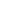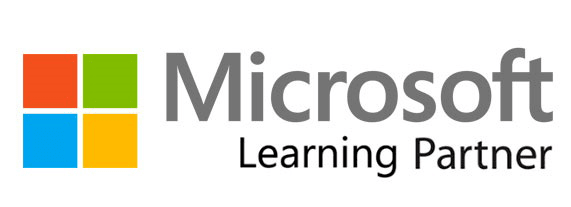18756 Stone Oak Park Way, Suite200, San Antonio TX 78258 USA
100 Queen St W, Brampton, ON L6X 1A4, CanadaUnited States# MB-310T00: Microsoft Dynamics 365 Finance Training

###### What MB-310T00: Microsoft Dynamics 365 Finance training is all about?

With this 4-day training, students will learn about various financial components of Microsoft Dynamics 365: configuring and using key financial components, accounts receivable, fixed assets, budgeting, collections, accounts payable, and more.

This training is based on the objectives of the course variant MB-310T100-A.

### 4 SATVs applicable

###### Schedule
• Delivery Format:
•Date: Nov 16, 2020 | 9:00 am - 5:00 pm EST
Location: Online
\$2300 USD
• Delivery Format:
•Date: Dec 14, 2020 | 9:00 am - 5:00 pm EST
Location: Online
\$2300 USD
###### What are the course objectives for MB-310T00: Microsoft Dynamics 365 Finance training?
• Understanding and performing financial duties in Dynamics 365 Finance
###### Who should attend MB-310T00: Microsoft Dynamics 365 Finance training?

A Dynamics 365 for Finance and Operations Functional Consultant is responsible for configuring applications and solutions, determining, and translating requirements, discovering, engaging stakeholders and subject matter experts. They are also responsible for implementing a suitable solution using service and application integrations, codeless extensibility, and unique capabilities.

###### What is the course outline for MB-310T00: Microsoft Dynamics 365 Finance training?
• 1. Financial Management
• a). Benefits of financial management
• b). Financial management
• c). Module summary
• 2. Configure Currencies
• a). Introduction
• b). Setup legal entity currencies and conversions
• c). Configure and import currency exchange rates
• d). Practice lab
• e). Module summary
• 3. Create Fiscal Calendars, Years and Periods
• a). Introduction
• b). Create fiscal calendars, years and periods
• c). Create date intervals
• d). Practice lab
• e). Module summary
• 4. Configure Chart of Accounts
• a). Introduction
• b). Define and configure the chart of accounts
• c). Define and configure the financial dimensions
• d). Define and configure account structures and rules
• e). Practice labs
• f). Module summary
• 5. Configure Ledger and Journals
• a). Journals and their setup
• b). Configure ledger and journal setup
• c). User journals
• d). Practice lab
• e). Module summary
• 6. Configure Cash and Bank Management
• a). Introduction
• b). Bank transaction types and groups
• c). Setup cash and bank management parameters
• d). Configure banks and bank checks
• e). Deposit and refund checks
• f). Cash flow
• g). Practice Labs
• h). Module summary
• 7. Use Cash and Bank Management
• a). Make deposits and perform payment reversals
• b). Use cash and bank management workspaces
• c). Practice Labs
• d). Module summary
• 8. Configure Letters of Credit and Guarantees
• a). Introduction
• b). Configure letters of credit
• c). Configure letters of guarantee
• d). Module summary
• 9. Perform Daily Procedures
• a). Learn about general ledger processes
• b). Create voucher templates
• c). Setup journal controls
• d). Practice Lab
• e). Module summary
• 10. Intercompany Accounting
• a). Setup and use intercompany accounting
• b). Practice Lab
• c). Module Summary
• 11. Configure Ledger Allocations and Accruals
• a). Introduction
• b). Configure and apply accrual schemes
• c). Configure ledger allocation rules
• d). Practice labs
• e). Module summary
• 12. Configure Sales Tax
• a). Understand tax engine components
• b). Practice lab
• c). Module summary
• 13. Configure Accounts Payable
• a). Understand Task recorder functionality
• b). Configure accounts payable parameters
• c). Create and maintain vendors
• d). Configure accounts payable charges
• e). Configure and use positive pay
• f). Practice Lab
• g). Module Summary
• 14. Perform Accounts Payable Daily Procedures
• a). Introduction
• b). Process orders, invoices, and payments
• c). Manage and apply prepayments
• d). Practice Labs
• e). Module summary
• 15. Use Accounting Distribution and Invoice Validations
• a). Introduction
• b). Settle transaction and undo settlements
• c). Distribute funds
• d). Configure invoice validation policies
• e). Practice labs
• f). Module summary
• 16. Configure Accounts Receivable
• a). Configure accounts receivable
• b). Create and maintain customers
• c). Setup customer posting profiles
• d). Configure accounts receivable charges
• e). Practice Lab
• f). Module summary
• 17. Perform Accounts Receivable Daily Procedures
• a). Create free text invoices and record customer payments
• b). Settle transactions and undo settlement
• c). Practice Lab
• d). Module summary
• 18. Configure Credit and Collections
• a). Introduction
• b). Configure credit and collections components
• c). Setup and manage interest
• d). Waive, reinstate or reverse
• e). Setup and manage collection letters
• f). Practice Lab
• g). Module Summary
• 19. Process Credit and Collections
• a). Introduction
• b). Understand how to process collections
• c). Manage customer credit and collections
• d). Practice Labs
• e). Module Summary
• 20. Configure PostDated Checks
• a). Introduction
• b). Manage post dated checks
• c). Practice Labs
• d). Module Summary
• 21. Configure and Use Basic Budgeting and Budget Control Processes
• a). Introduction
• b). Configure basic budgeting components
• c). Configure budget control components
• d). Create and test budget registry entries
• e). Use inquiries and reports
• f). Practice Labs
• g). Module summary
• 22. Configure and Use Budget Planning Processes
• a). Introduction
• b). Configure budget plannign components
• c). Practice Lab
• d). Module summary
• 23. Configure Fixed Assets Management
• a). Introduction
• b). Configure fixed asset components
• c). Enable fixed asset integration
• d). Create fixed assets
• e). Practice labs
• f). Module summary
• 24. Manage Fixed Assets
• a). Introduction
• b). Perform fixed assets tasks
• c). Practice labs
• d). Module summary
• 25. Configure and Use Cost Accounting
• a). Introduction
• b). Cost control workspace
• c). Practice lab
• d). Module summary
• 26. Configure and Use Periodic Processes
• a). Introduction
• b). Bank reconciliations
• c). Configure financial period close
• d). Prepare periodic filings
• e). Run foreign currency revaluation processes
• f). Perform financial consolidations
• g). Process end of year close
• h). Practice Labs
• i). Module summary
• 27. Configure the Electronic Reporting Framework
• a). Introduction
• b). Configure electronic reporting
• c). Formula designer in electronic reporting
• d). Practice Lab
• 28. Configure Localized Features
• a). Introduction
• b). Localization and regulatory features
• c). Classification of Mexican localization features
• d). Understand the global tax engine
• e). Understand the features of fiscal establishments and documents for Brazilian localization
• f). Bill of Exchange
• g). Electronic Invoices
• h). Practice Labs
• i). Module summary
• 29. Configure Financial Management for Public Sector
• a). Introduction
• b). Setup funds for the public sector
• c). Setup derived financial hierarchies